# Derivation of the Transfer Functions for Error Amplifiers, Voltage Amplifiers, Current Amplifiers

2016.11.24

・It is confirmed that general transfer functions for a voltage amplifier and a current amplifier can be derived from the derived transfer function for an error amplifier.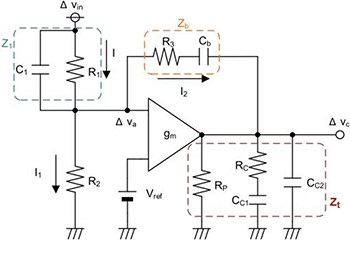Fig. 1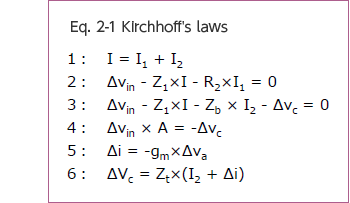## Transfer function of an error amplifier

Figure1 is an example of a general error amplifier block. This amplifier compares Vin and Vref, and outputs the results as Vc. Here we derive the transfer function for this amplifier, but first, confirm for yourself that Z1, Zb and Zt are as follows for the circuit blocks surrounded by dashed lines in the diagram.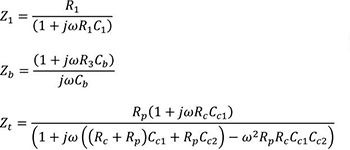Next, Kirchhoff’s laws are used to derive the transfer function (Δvc/Δvin) for the amplifier of Fig. 1.

The Kirchhoff laws used in the derivation are shown in eq. 2-1.

Next, the transfer function derived using eq. 2-1 is shown in eq. 2-2.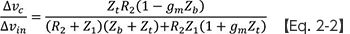Eq. 2-2 can be treated as a general equation for the transfer function of an error amplifier such as that of Fig. 1. Below, eq. 2-2 is used to examine the transfer functions of “a voltage amplifier” and “a current amplifier”, which are essential when considering DC-DC converters.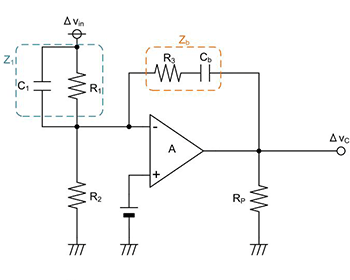Fig. 2

## Transfer function of a voltage amplifier

Fig. 2 shows an example of a voltage amplifier configuration. Eq. 2-3 below is a general relationship, appearing on data sheets and the like, for the transfer function of a voltage amplifier.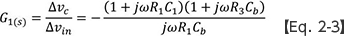We check whether we can derive this eq. 2-3 using the previously derived eq. 2-2. The conditions for eq. 2-2 are as follows.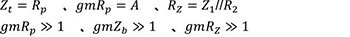Modifying eq. 2-2 based on these conditions, we arrive at eq. 2-4.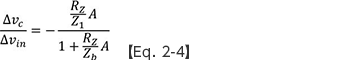Here, when eq. 2-4 satisfies the conditions of eq. 2-5 below, eq. 2-4 can be expressed as eq. 2-6: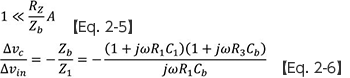Compare this eq. 2-6 with eq. 2-3 above, presented as a general equation for the transfer function of a voltage amplifier. You should be able to see that the two are equivalent.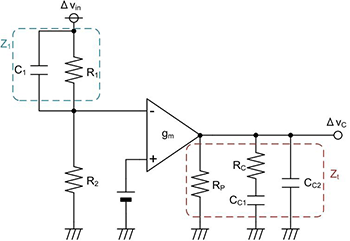Fig. 3

## Transfer function of a current amplifier

Next, Fig. 3 shows an example of a current amplifier configuration. Eq. 2-7 below is likewise the equation generally used for the transfer function of this amplifier.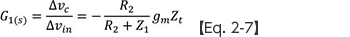Now we similarly attempt to derive eq. 2-7 using eq. 2-2. The conditions for eq. 2-2 are as follows.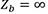Modifying eq. 2-2 based on this, we can derive the following eq. 2-8.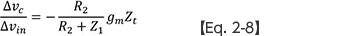Upon comparison with eq. 2-7, it should again be clear that the two are equivalent.

Thus it is seen that transfer functions for a voltage amplifier and a current amplifier can be derived from the transfer function for the error amplifier of Fig. 1 derived in the beginning.